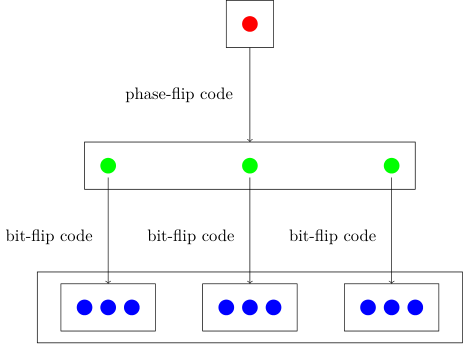# The Shor Code#

It is now time to construct a code that correct more than one type of error. This will also give us the opportunity to show off a code construction technique called code concatenation.

In code concatenation, we first encode the logical qubit using a particular code into $n$ physical qubits. Then, we encode each of then $n$ physical qubits individually using the same or another code. To illustrate this, recall that, in the phase-flip code the logical basis states are encoded as \begin{align} \ket{0} \to \ket{\bar{0}} = \ket{+++}, \\ \ket{1} \to \ket{\bar{1}} = \ket{---}. \end{align} Now, we are going to take each of the three physical qubits and encode them using the bit-flip repetition code. Recall that in the bit-flip repetition code, the plus and minus states encode as \begin{align} \ket{+} &= \frac{\ket{0} + \ket{1}}{\sqrt{2}} \to \ket{\bar{+}} = \frac{\ket{000} + \ket{111}}{\sqrt{2}}, \\ \ket{-} &= \frac{\ket{0} - \ket{1}}{\sqrt{2}} \to \ket{\bar{-}} = \frac{\ket{000} - \ket{111}}{\sqrt{2}}. \end{align} When we apply this encoding to each of the three qubits in the phase-flip encoding, we obtain \begin{align} \ket{+++} \to \ket{\bar{\bar{0}}} = \frac{(\ket{000} + \ket{111})(\ket{000} + \ket{111})(\ket{000} + \ket{111})}{2\sqrt{2}}, \\ \ket{---} \to \ket{\bar{\bar{1}}} = \frac{(\ket{000} - \ket{111})(\ket{000} - \ket{111})(\ket{000} - \ket{111})}{2\sqrt{2}}. \end{align} Visually, we can draw a tree diagram to show how one qubit is first encoded into three via the phase-flip code and then each of the three qubits is encoded into three more via the bit-flip code.Here we refer to the phase-flip code as the outer code (level 1) and the bit-flip code as the inner code (level 2).

### Encoding#

This combined action that maps one logical qubit to the state of nine qubits as \begin{align} \ket{0} \to \ket{\bar{\bar{0}}} = \frac{(\ket{000} + \ket{111})(\ket{000} + \ket{111})(\ket{000} + \ket{111})}{2\sqrt{2}}, \\ \ket{1} \to \ket{\bar{\bar{1}}} = \frac{(\ket{000} - \ket{111})(\ket{000} - \ket{111})(\ket{000} - \ket{111})}{2\sqrt{2}}, \end{align} defines the encoding for the Shor code.

Create the encoding circuit for the Shor code. You will use nine qubits.

1. Apply the phase-flip repetition code's encoding circuit to qubits 0, 3, 6.
2. Apply the bit-flip repetition code's encoding circuit to
• qubits 0,1,2
• qubits 3,4,5
• qubits 6,7,8

Then use the circ.simulate() method to check if you get the correct output.

import stac
enc_circ = stac.Circuit()


Question: Construct the basis states of a nine-qubit code with the bit-flip code as the outer code and the phase-flip code as the inner code.

### Errors and decoding#

We will now show that this code can correct every possible single-qubit error. Let's first show that it can correct bit-flip and phase-flip errors on any of the nine physical qubits.

#### $X$-errors#

Suppose a single-qubit $X$ error occurs, on say the fourth qubit. \begin{align} X_4\ket{\bar{\bar{0}}} = \frac{(\ket{000} + \ket{111})(\ket{010} + \ket{101})(\ket{000} + \ket{111})}{2\sqrt{2}}, \\ X_4\ket{\bar{\bar{1}}} = \frac{(\ket{000} - \ket{111})(\ket{010} - \ket{101})(\ket{000} - \ket{111})}{2\sqrt{2}}, \end{align} How can one detect that this occur occured? We will do the following syndrome measurements, in the style of the bit-flip code.

• Compare the values of qubits 0,1,2
• Compare the values of qubits 3,4,5
• Compare the values of qubits 6,7,8

In this example, comparing the value of the 3rd qubit with the 4th, and the 4th with the 5th will show that the 3rd qubit has a different value. The error can be fixed as before.

Create the syndrome measurement circuit for detecting $X$ errors in the Shor code.

sync_circ_x = stac.Circuit()


#### $Z$-errors#

The case for a single-qubit $Z$ errors is slightly more difficult to see. Note, for instance, that if a $Z$ error occurs on any one of the first three qubits, the sign in the first block will change. For $i = 1,2,3$, \begin{align} Z_i\ket{\bar{\bar{0}}} = \frac{(\ket{000} - \ket{111})(\ket{000} + \ket{111})(\ket{000} + \ket{111})}{2\sqrt{2}}, \\ Z_i\ket{\bar{\bar{1}}} = \frac{(\ket{000} + \ket{111})(\ket{000} - \ket{111})(\ket{000} - \ket{111})}{2\sqrt{2}}. \end{align} A different way of seeing it is at the outer code level, where the encoding is \begin{align} \ket{\bar{\bar{0}}} = \ket{\bar{+}}\ket{\bar{+}}\ket{\bar{+}}, \\ \ket{\bar{\bar{1}}} = \ket{\bar{-}}\ket{\bar{-}}\ket{\bar{-}}. \end{align} Then the action of $Z_i$ for $i=0,1,2$ is \begin{align} Z_i\ket{\bar{\bar{0}}} = \ket{\bar{-}}\ket{\bar{+}}\ket{\bar{+}}, \\ Z_i\ket{\bar{\bar{1}}} = \ket{\bar{+}}\ket{\bar{-}}\ket{\bar{-}}. \end{align} At this level, it is quite easy to see the error-detecting strategy. Apply the phase-flip code error-detection strategy to the three encoded qubits (at the outer level). We will discuss how one can do this later.

#### $Y$-errors#

Right now, we want to show that the Shor code can detect and correct errors beyond just $X$ and $Z$ errors. One such error is the $Y$ error, which is just $Y = \iu ZX$, i.e. a combined bit-flip and phase-flip error. For instance, $Y_4$ will result in the corrupted basis states \begin{align} Y_4\ket{\bar{\bar{0}}} = -\iu \frac{(\ket{000} + \ket{111})(\ket{010} - \ket{101})(\ket{000} + \ket{111})}{2\sqrt{2}}, \\ Y_4\ket{\bar{\bar{1}}} = -\iu \frac{(\ket{000} - \ket{111})(\ket{010} + \ket{101})(\ket{000} - \ket{111})}{2\sqrt{2}}, \end{align}

This error is detected at both the $X$ detection stage, and the $Z$ detection stage, and corrected at both as well. First, we detect $X$ errors, find that the fourth is flipped and fix it. Then we detect $Z$ errors and determine that the middle block has experienced an error and fix it.

Hence, we have shown up till now that the Shor code can correct all errors in the set, \begin{equation} \st{E} = I \union \set{X_i}_i \union \set{Y_i}_i \union \set{Z_i}_i, \quad i = 0,\dots,8. \end{equation}

#### Unitary errors#

We showed before that the bit-flip code could also correct for $X$ rotation errors. By the same arguments of linearity and collapse, the Shor code can correct any unitary error which is of the form, \begin{equation} E_i = e_0 I + e_1X_i + e_2 Y_i + e_3Z_i, \quad \sum_j|e_j|^2 = 1. \end{equation}

Apply $E_i$ to $\ket{\bar\psi}$ and then show that the error-detection circuit will collapse the state to just one of the possible errors.
Question: Suppose the state $\ket{\bar\psi}$ encoded by the Shor code undergoes the two-qubit error $Z_1Z_2$. What is the impact of this error? This is a phenomena not seen in classical codes.